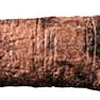#### You may also like### Babylon Numbers

Can you make a hypothesis to explain these ancient numbers?### Ishango Bone

Can you decode the mysterious markings on this ancient bone tool?### White Box

This game challenges you to locate hidden triangles in The White Box by firing rays and observing where the rays exit the Box.

# Black Box

##### Age 16 to 18 Challenge Level:

Well done to Patrick of Woodbridge School, Ben, Lower 6 at Kingston Grammar, William of Bishop Wordsworth's School and Herschel of the European School of Varese who all sent in correct solutions to this very difficult problem, showing the great tenacity and determination of the most mathematically minded! We love the way the journeys, with their ups and downs, are described in these solutions.

Before putting in the full solutions, special mention must be given to Madeline aged 10 from St. Josephs who discovered that the first function gave the prime numbers. She said very clearly:

left numbers = y,  right numbers = x,  y = xth prime number

This is how Lower 6 at Kingston Grammar tackled the problem:

Function 1: First we looked for an algebraic solution. We tried multiplicative and additive rules and a combination of both but none worked. We quickly realised that this was not the case. Then Freddie suspected that all the outputs were prime. So we tried nearest prime to a multiple of the input but this didn't work either. It became easier when we looked at the lower inputs. Then Owen had an epiphany in a moment of pure inspiration that
$f(x) =$ the $x^{th}$ prime!
We had solved the puzzle in our class as a team.

For function 2, we spent a while looking at the numbers generated seeing nothing in particular except the fact that prime numbers were always one less than themselves. This prompted us to look at factors. After a long while thinking about factors and minusing one, we looked at prime factors. This is when Andrew found the rule.
$f(x) =$ the product of all unique prime factors each minus one multiplied by the other prime factors without minusing one.

For example, $60 \to 16$ since $60 = 2\times 2\times 3\times 5$ and therefore $(2-1)\times(3-1)\times(5-1)\times2=16$.
$2,3,5$ are the unique factors reduced by one, then the $2$ is multiplied without being reduced by one.

For function 3, we were again stumped for quite a while. We realised in this case that there was a clearer relationship between $x$ and $f(x)$. First we tried $y=mx+c$. This didn't work, so we plotted a few points. Richard noticed that it looked a lot like the graph of $y = ln x$. This reminded him of Gauss's approximation for the distribution of the primes below any number. (Which was in fact $x/(lnx)$.) It seemed to fit Gauss's formula pretty well and after a few checks it was obvious that this was true. So $f(x)=$ number of primes up to x .

After deducing function 2, Herschel remarked that "the business of only reducing each distinct factor once was an evil trick!" Tricky it may have been but an evil trick it is not. Patrick correctly identified this as Euler's totient function which gives the number of numbers less than n which are coprime to n. It is a particularly useful function especially within public key cryptography.

Ben worked out function 4 and 5 for us:

Function 4: This one I didn't need to plot a graph for as I knew it was going to be (kind of) about primes. So again I got a small sample and looked at the primes that made up each value of x and looked for a pattern between them and y. Very quickly I noticed that y was the number of numbers that go into x is divisible by. So I think that function 4 returns the number of numbers that go into x that are below or equal to x.

Function 5: Now this was a hard one but I managed to crack it. I started off looking at the connection with primes again but this went nowhere, and nor did plotting a graph, so I decided to try and get the first 10 values (this took me ages!) as these are easier to analyse than bigger numbers, and I still thought that it might have something to do with primes. This function on the other hand has nothing to do with primes, and the first 5 values of y are $3,1,4,1,5$ which you can clearly see are the first 5 digits of pi (well I could because I know the first 11 by heart $\ddot\smile$). Checking this for larger values of x showed that it worked. It also helped explain why each y value was only 1 digit in length. So function 5 returns the $n^{th}$ digit of pi.

Patrick showed great persistance in his solution:

Function 5: I soon found that $78$ appeared to be one of a very few numbers outputting $0$. I consulted Wolfram|Alpha to investigate properties of $78$, but nothing seemed to be obvious. It was a very bizarre function; at one point, I thought the graph of $x$ against $y$ was a message, since so many values overlapped. Given that all the values were below $10$, I tried thinking of modular functions to model this. The value $(1,3)$ was particularly problematic; $1$ mod anything is $1$. I spent about $30$ minutes on it, finding the first $15$ values, before I read down the column: $3-4-5926535$ This is just the decimal places of pi, and is thus random. The whole problem took me two hours, but I got there in the end!